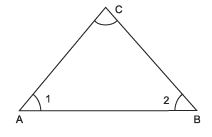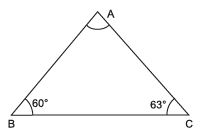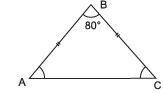Courses

# Very Short Answer Type Questions- Triangles Class 9 Notes | EduRev

## Class 9 Mathematics by Full Circle

Created by: Full Circle

## Class 9 : Very Short Answer Type Questions- Triangles Class 9 Notes | EduRev

The document Very Short Answer Type Questions- Triangles Class 9 Notes | EduRev is a part of the Class 9 Course Class 9 Mathematics by Full Circle.
All you need of Class 9 at this link: Class 9

Question 1. In a ΔABC, BC = CA and ∠ A = 50°, which is longer BC or AB?
Solution:
∵ ∠ 1 = ∠ 2  [∵ AC = BC]
and ∠A = ∠1 = 50°⇒ ∠ 2 = 50°
∴ ∠ C = 180º - [50° + 50°] = 80°
Since, ∠C > ∠A
⇒ AB > BC Thus, AB is greater.

Question 2. In ΔABC, ∠ B = 60° and ∠ C = 63°. Name the greatest side.
Solution:
In ΔABC, ∠ B = 60° and ∠ C = 63°∠ A = 180º - (60° + 63°) = 57°
⇒ The greatest side is opp. to the greatest angle i.e., 63°
∴ Side AB is the greatest.

Question 3. In ΔABC, if BC = AB and ∠ B = 80° then find the measure of∠ A.
Solution:
BC = AB ⇒ ∠A = ∠C∵ ∠B = 80°
∴ ∠A + ∠C = 180° - 80° = 100°
⇒ ∠A = ∠C = 50º

Question 4. Which of the following is not the criterion for congruence of D s? (i) SAS, (ii) SSA, (iii) ASA, (iv) RHS
Solution:
SSA is not the criterion for congruency.

Question 5. If two angles are (30 ∠ a)º and (125 + 2a)º and they are supplement of each other. Find the value of ‘a’.
Solution:
∵ (30 - a)º and (125 + 2a)º are supplement to each other.
∴ (30 - a + 125 + 2a)º = 180º
⇒ a = 180º - 125º - 30º = 25º
⇒ Value of a = 25°

Question 6. Each of the equal angles of an isosceles triangle is 38°, what is the measure of the third angle?
Solution:
Let the third angle = x
∴ x + 38° + 38° = 180°

⇒ x = 180° - 38° - 38°
= 104º

Question 7. In an isosceles ΔABC, AB = AC and ∠ A= 80°. What is the measure of ∠ B?
Solution:
We have AB = AC ⇒ ∠ B = ∠ C          [∵ Angles opposite to equal sides are equal]
∴ ∠A + ∠B + ∠C = 180°
⇒ ∠A + ∠B + ∠B = 180°
⇒ ∠A + 2∠B = 180°
⇒ 80° + 2∠B = 180°
⇒ 2∠B = 180°- 80°
= 100
⇒ ∠B= (100o/2) = 50°

Question 8. Find the measure of each of acute angle in a right angle isosceles triangle.
Solution:
Let the measure of each of the equal acute angle of the Δ be x
∴ We have: x + x + 90° = 180°
⇒ x + x = 180° - 90° = 90°
⇒ x= (90o/2)= 45°

,

,

,

,

,

,

,

,

,

,

,

,

,

,

,

,

,

,

,

,

,

;